Courses

# Mohr's Circle For Plane Stress & Plane Strain Notes | EduRev

## Mechanical Engineering : Mohr's Circle For Plane Stress & Plane Strain Notes | EduRev

The document Mohr's Circle For Plane Stress & Plane Strain Notes | EduRev is a part of the Mechanical Engineering Course Strength of Materials (SOM).
All you need of Mechanical Engineering at this link: Mechanical Engineering

Mohr’s circle is the locus of points representing the magnitude of normal and shear stress at the various plane in a given stress element. Graphically, a variation of normal stress and shear stress are studied with the help of Mohr's circle.
σ1 and σ2 are Principal Stresses,  then normal and shear stress on lane  which is inclined at angle ‘θ’ from major principal plane, then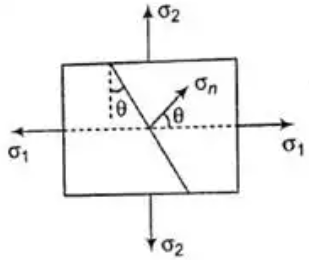Different Stress Diagram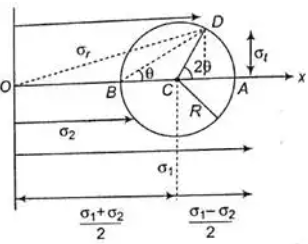Mohr's circle for plane Stress and StrainNormal Stress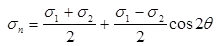Shear Stress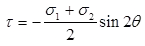General State of Stress at an Element

If  σx and σy are normal stress on vertical and horizontal plane respectively and this plane is  accompanied by shear stress  then normal stress and shear stress on the plane, which is inclined at an angle θ from the plane of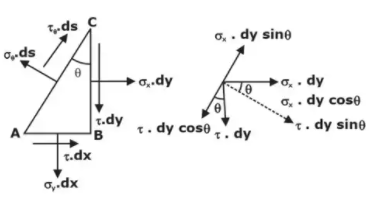then,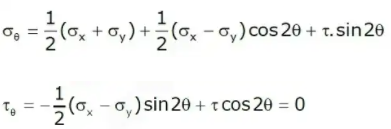Let σx, σy be two normal stresses(both tensile) and τxy be shear stress then:

Maximum and Minimum Principal Stresses are: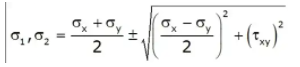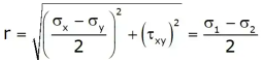Strength of Materials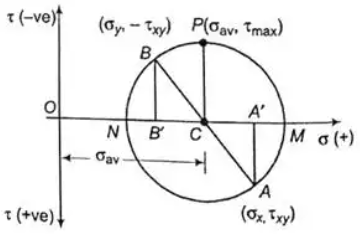Mohr's circle for plane stressed

Observations from Mohr's Circle

The following are the observations of Mohr's circle as:

• At point M on circle σn is maximum and shear stress is zero.
∴ Maximum principal stress ≡ coordinate of M
• At point N on circle, σn is minimum and shear stress τ is zero.
∴ minimum principal stress ≡ coordinate of N
• At point P on Circle τ is maximum.
Maximum shear stress ≡ ordinate of P(i.e. radius of circle)
• Also, normal stress on the plane of maximum shear stress:
σn = abscissa of P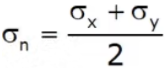Where σn ≡ Average stress
• Mohr's circle becomes zero at a point if radius of circle has the following consideration.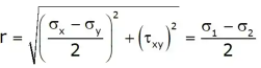• If σx = σy, then radius of Mohr's circle is zero and τxy = 0.
• The sum of normal stresses acting on perpendicular faces of a plane stress elements is constant and independent of the angle θ.
σ1 + σ2 = σ1 + σ2

Strain analysis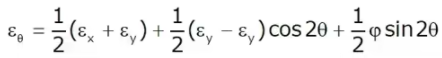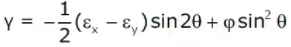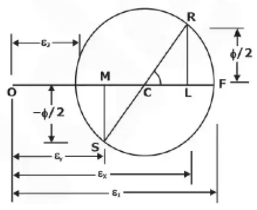Principle Strain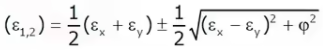θ + ∈θ + 90º = ∈x + ∈y

Relation between Principle strain and stress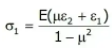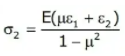Strain Rosetts

• Rectangular Strain Rosette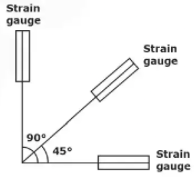x = ∈, ∈y = ∈90º and Ф = 2∈45º - (∈x + ∈y)

• Equiangular Strain Rosette (delta strain rosette)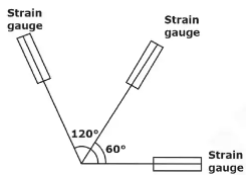θº = x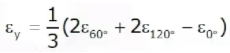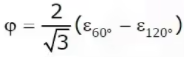Offer running on EduRev: Apply code STAYHOME200 to get INR 200 off on our premium plan EduRev Infinity!

## Strength of Materials (SOM)

35 videos|33 docs|48 tests

,

,

,

,

,

,

,

,

,

,

,

,

,

,

,

,

,

,

,

,

,

;